• img1) cv2.waitKey(0) cv2.destroyAllWindows() 由于OpenCV中的通道为B G R ，所以0代表B通道，以此类推，代码中设B和R通道为0，那么图像就是绿色了。实验效果： 赵敏小姐姐，哈哈。 来张红色的： import cv2 import...
直接上代码：
import cv2
import  matplotlib.pyplot as plt
import  numpy as np

# img = img[0:500,0:2000]
b,g,r = cv2.split(img)
img1 = img.copy()
img1[:,:,0] = 0
img1[:,:,2] = 0
cv2.imshow("G",img1)
cv2.waitKey(0)
cv2.destroyAllWindows()
由于OpenCV中的通道为B G R ，所以0代表B通道，以此类推，代码中设B和R通道为0，那么图像就是绿色了。实验效果：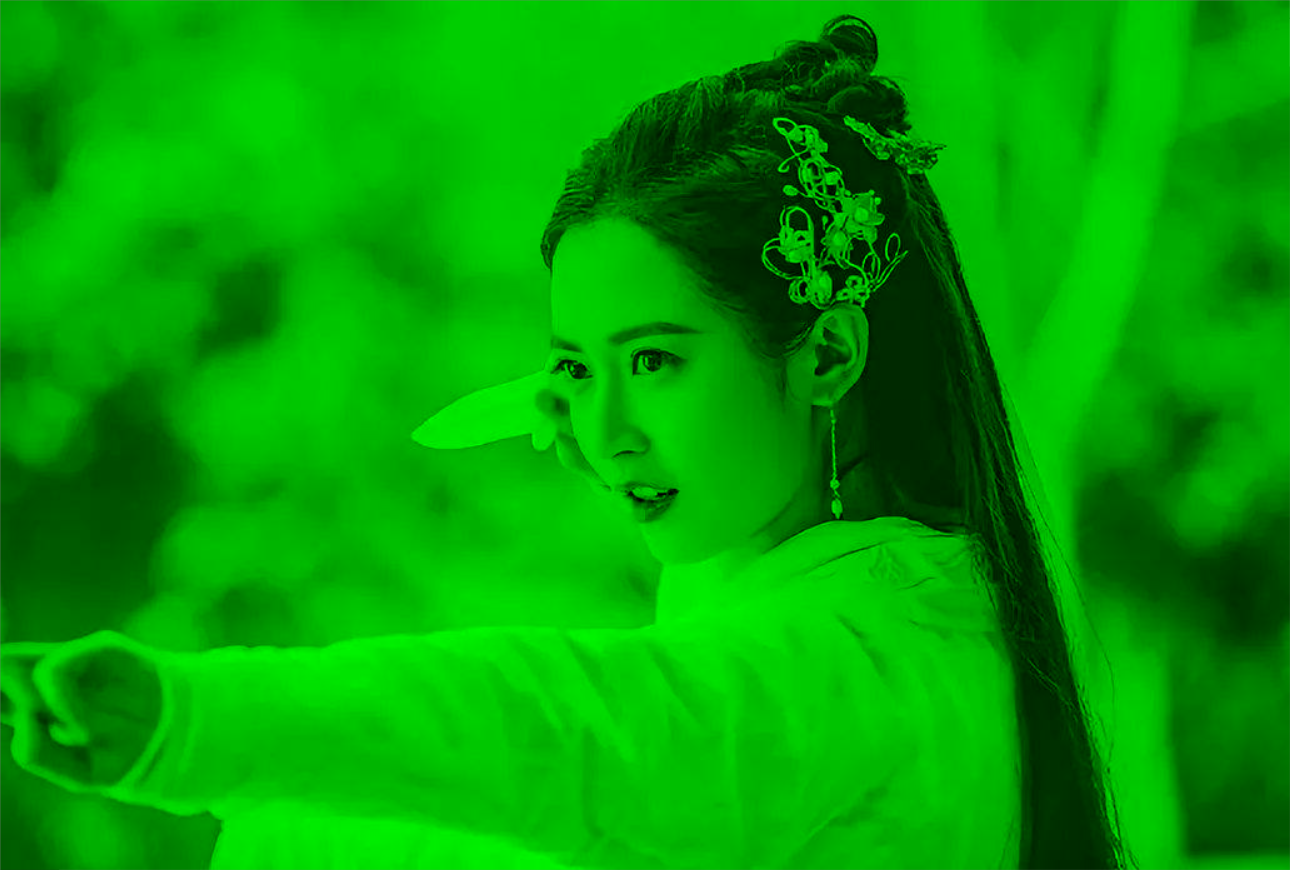赵敏小姐姐，哈哈。
来张红色的：
import cv2
import  matplotlib.pyplot as plt
import  numpy as np

# img = img[0:500,0:2000]
b,g,r = cv2.split(img)
img1 = img.copy()
img1[:,:,0] = 0
img1[:,:,1] = 0
cv2.imshow("R",img1)
cv2.waitKey(0)
cv2.destroyAllWindows()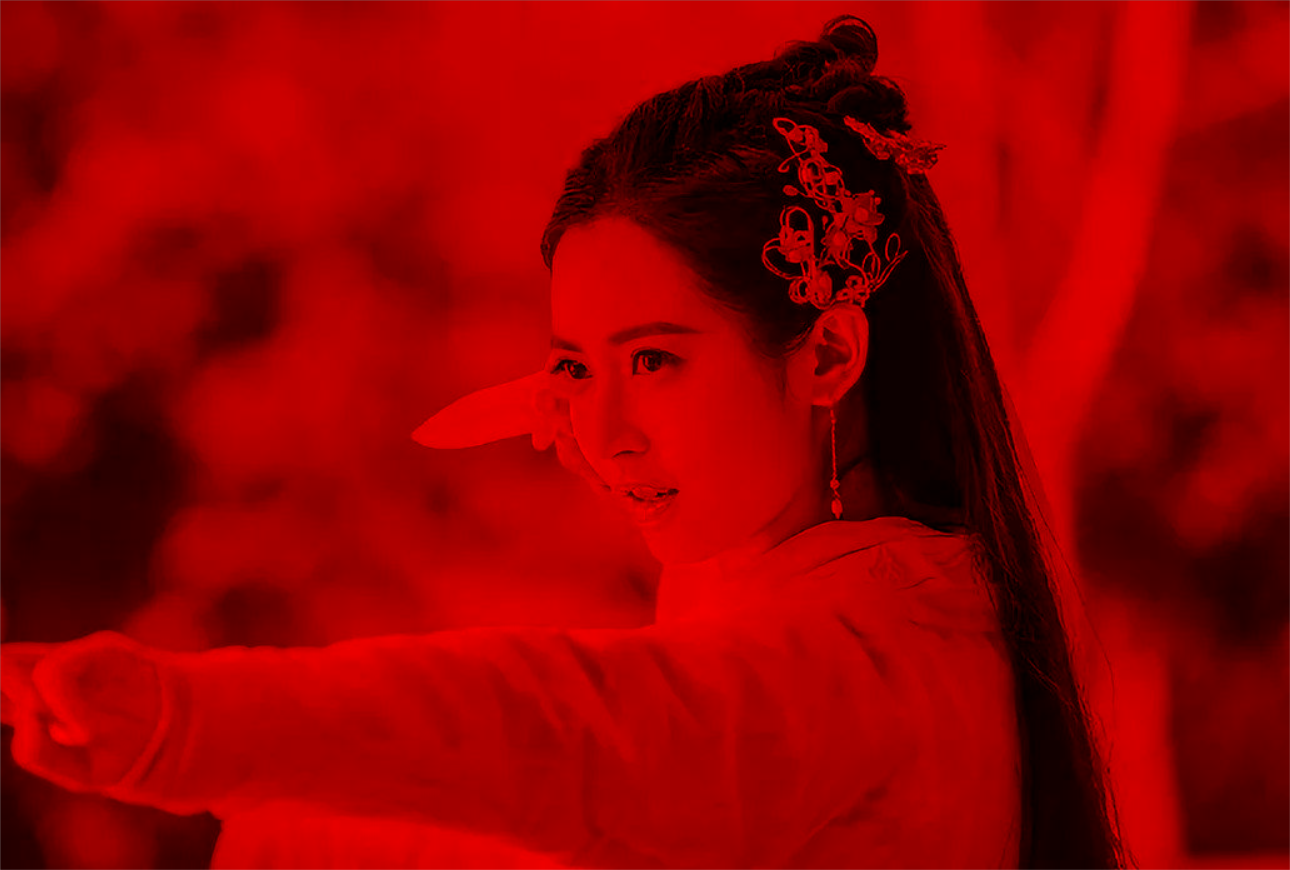展开全文• plt.imshow(img[:,:,2],plt.cm.gray) #绘制第四幅图片,且为灰度图 plt.axis('off') #不显示坐标尺寸 # plt.show() # img=data.astronaut() hsv = color.rgb2hsv(img) # # fig, axes = plt.subplots(2, 2, figsize=(7...
这里调用里面自带的库 头文件列表如下：
from skimage import data from skimage import data,color import matplotlib.pyplot as plt
from skimage import data
from skimage import data,color
import matplotlib.pyplot as plt

img=data.astronaut()
plt.figure(num='astronaut',figsize=(8,8))  #创建一个名为astronaut的窗口,并设置大小

plt.subplot(2,4,1)     #将窗口分为两行两列四个子图，则可显示四幅图片
plt.title('origin image')   #第一幅图片标题
plt.imshow(img)      #绘制第一幅图片

plt.subplot(2,4,2)     #第二个子图
plt.title('R channel')   #第二幅图片标题
plt.imshow(img[:,:,0],plt.cm.gray)      #绘制第二幅图片,且为灰度图
plt.axis('off')     #不显示坐标尺寸

plt.subplot(2,4,3)     #第三个子图
plt.title('G channel')   #第三幅图片标题
plt.imshow(img[:,:,1],plt.cm.gray)      #绘制第三幅图片,且为灰度图
plt.axis('off')     #不显示坐标尺寸

plt.subplot(2,4,4)     #第四个子图
plt.title('B channel')   #第四幅图片标题
plt.imshow(img[:,:,2],plt.cm.gray)      #绘制第四幅图片,且为灰度图
plt.axis('off')     #不显示坐标尺寸
# plt.show()

# img=data.astronaut()

hsv = color.rgb2hsv(img)
#
# fig, axes = plt.subplots(2, 2, figsize=(7, 6))
# ax0, ax1, ax2, ax3 = axes.ravel()

plt.subplot(2,4,5)
plt.imshow(img)
plt.title("Original image")
# plt.show()

# plt.subplot(2,4,5)
# ax0.imshow(img)
# ax0.set_title("Original image")

# plt.subplot(2,4,6)
# ax1.imshow(hsv[:, :, 0], cmap=plt.cm.gray)
# ax1.set_title("H channel")
plt.subplot(2,4,6)
plt.imshow(hsv[:, :, 0], cmap=plt.cm.gray)
plt.title("H channel")
# plt.show()

plt.subplot(2,4,7)
plt.imshow(hsv[:, :, 1], cmap=plt.cm.gray)
plt.title("S channel")

plt.subplot(2,4,8)
plt.imshow(hsv[:, :, 2], cmap=plt.cm.gray)
plt.title("V channel")

# for ax in axes.ravel():
#     ax.axis('off')
#
# fig.tight_layout()  #自动调整subplot间的参数

# fig, axes = plt.subplots(2, 4, figsize=(7, 6))
# ax0, ax1, ax2, ax3 = axes.ravel()
# ax0.imshow(img)
# ax0.set_title("Original image")
# plt.show()   #显示窗口

plt.show()   #显示窗口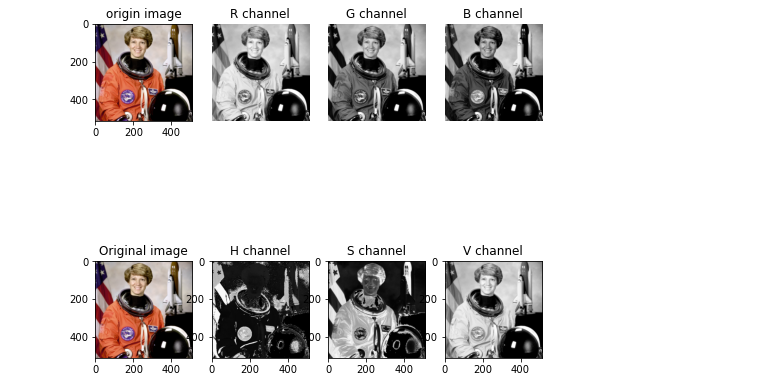参考原文链接： 链接：https://blog.csdn.net/dongbao520/article/details/115546978
展开全文opencv 计算机视觉 HSV
• 请参阅链接问题中的解决方案：您可以调整shift_hue()函数来调整色调,...原版的：def shift_hue(arr, hout):r, g, b, a = np.rollaxis(arr, axis=-1)h, s, v = rgb_to_hsv(r, g, b)h = houtr, g, b = hsv_to_rgb(h,...
请参阅链接问题中的解决方案：您可以调整shift_hue()函数来调整色调,饱和度和值,而不仅仅是调整色调.这应该允许您根据需要移动所有这些参数.
原版的：
def shift_hue(arr, hout):
r, g, b, a = np.rollaxis(arr, axis=-1)
h, s, v = rgb_to_hsv(r, g, b)
h = hout
r, g, b = hsv_to_rgb(h, s, v)
arr = np.dstack((r, g, b, a))
return arr
调整版本：
def shift_hsv(arr, delta_h, delta_, delta_v):
r, g, b, a = np.rollaxis(arr, axis=-1)
h, s, v = rgb_to_hsv(r, g, b)
h += delta_h
s += delta_s
v += delta_v
r, g, b = hsv_to_rgb(h, s, v)
arr = np.dstack((r, g, b, a))
return arr
假设您知道原始图像的基色和所需的目标颜色,则可以轻松计算增量：
base_h, base_s, base_v = rgb_to_hsv(base_r, base_g, base_b)
target_h, target_s, target_v = rgb_to_hsv(target_r, target_g, target_b)
delta_h, delta_s, delta_v = target_h - base_h, target_s - base_s, target_v - base_v
展开全文• 通过系统自带的图片处理进行转换HSV和RGB的单独划分通道图。 效果如图; 各个通道的数据分析图。 对应的源码1： import matplotlib.pyplot as plt from skimage import data,color img=data.astronaut() hsv = color...
通过系统自带的图片处理进行转换HSV和RGB的单独划分通道图。
效果如图;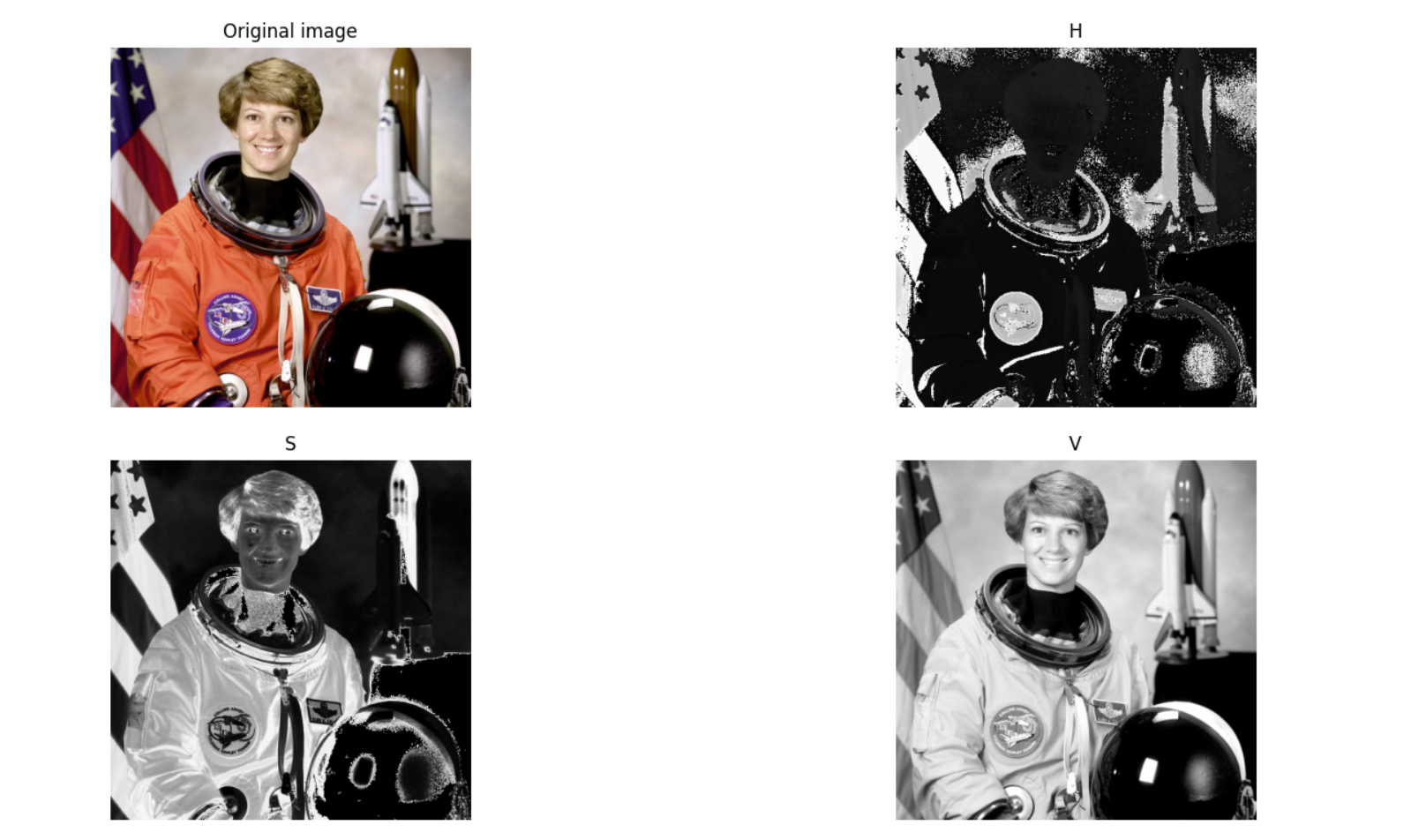各个通道的数据分析图。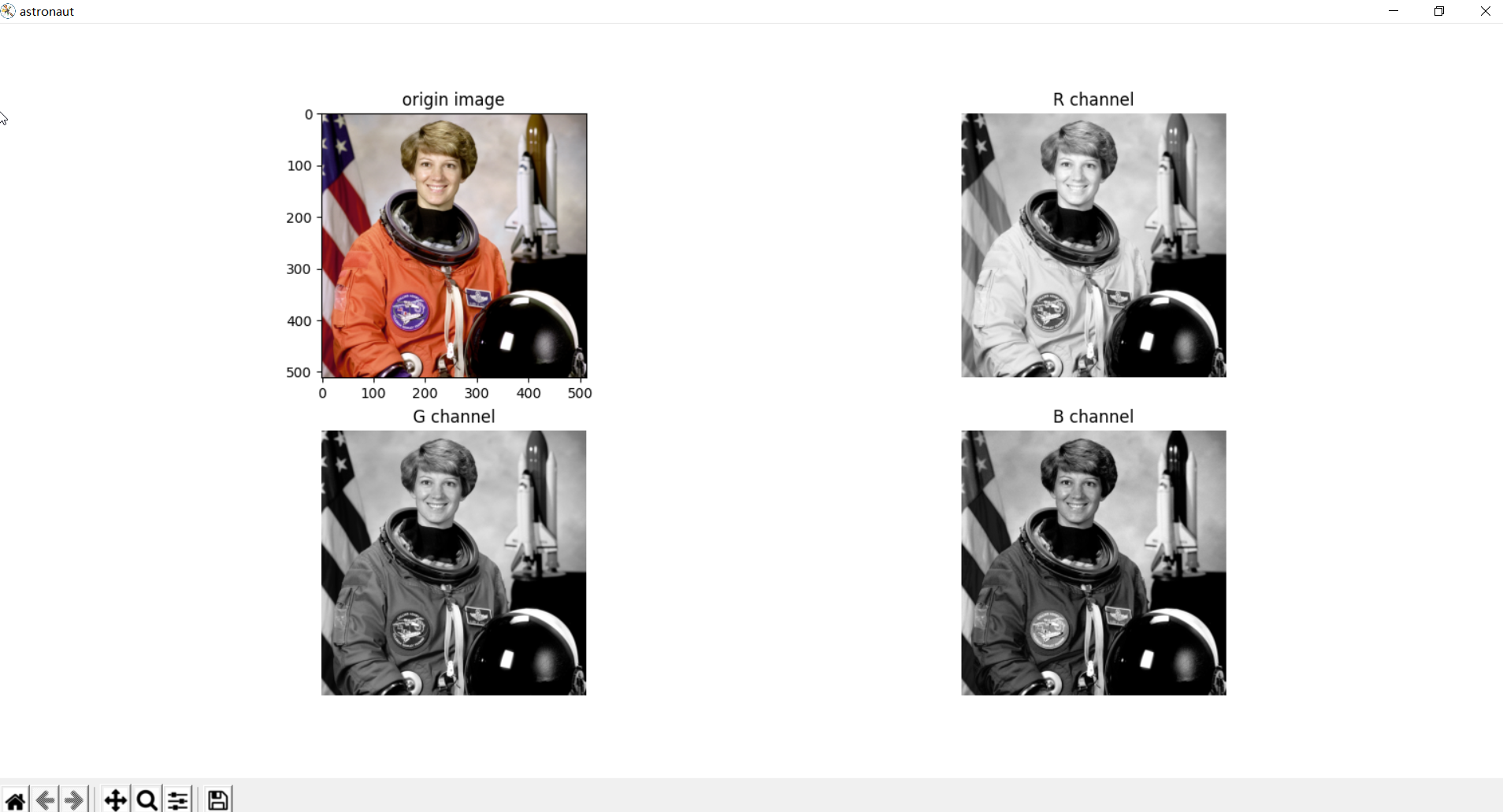对应的源码1：
import matplotlib.pyplot as plt
from skimage import data,color

img=data.astronaut()
hsv = color.rgb2hsv(img)</
展开全文• )b, g, r = cv2.split(img) #分离函数merged = cv2.merge([b,g,r]) #合并函数cv2.imshow('image',img)cv2.imshow("Blue 1", b)cv2.imshow("Green 1", g)cv2.imshow("Red 1", r)cv2....
• 本篇文章作为另一篇博文使用Python中OpenCV库创建一幅图片的RGB通道图片的补充。这篇博文中我们使用了数组切片及索引实现了分离BGR通道的功能。两种图层拆分方法今天，我们使用OpenCV提供的一组函数来实现相同的功能...
• ## RGB图像通道分离

千次阅读 2020-03-21 01:33:11
每一张彩色图片都可以由RGB3个通道组成，R指的是red红色，G指的是green绿色，B指的是blue蓝色。因此，可以将彩色图分离成三张图片，一张是红色，一张是绿色，一张是蓝色。 2，代码实现 import numpy as np ...
• How to split image to RGB colors and why doesn't split() function work?from PIL import Imagepil_image = Image.fromarray(some_image)red, green, blue = pil_image.split()red.show()Why does red.show() sho...
• python可以调用opencv中提供的诸多方法来完成，对图像像素访问，RGB通道分离与合并一. 像素访问 opencv中使用 imread读出来的图片直接可以通过 im[i,j,0] 访问i，j位置的R通道(默认是RGB) 代码示例：#访问像素...
• ## 【OpenCV】分离多通道图像RGB的值

万次阅读 多人点赞 2012-05-11 21:25:57
在《访问图像中每个像素的值》中我们介绍了存储图像的结构体...多通道字节/浮点型图像中存储了每个像素RGB三信道的值。 最近在做的项目中需要提取图像ROI区域RGB的平均值，发现有一个cvAvg函数： CvScalar cvAvg( codst 数据结构 存储 merge c#
• 2、从一副彩色图像分离出R、G、B三个通道（cvSplit），并且显示 3. 分别对每个通道图像创建直方图，并显示 首先介绍一下opencv中的cvSplit函数： 函数原型： [cpp] view ...opencv c++
• 图片组成 [ [[245 227 220] [245 226 219] [245 227 220] ...分离RGB值 def writeFile(X,filename): if filename!="": with open(filename,"w")as f: json.dump(X,f,indent=4) def getMatrixRGBopencv
• #OpenCV 中 imread() 函数默认加载图像文件，加载进来的是 3 通道彩色图像，色彩空间 是RGB色彩空间。 通道顺序是：BGR （蓝色、绿色、红色）。 通道分离函数：split（） 通道合并函数：merge（） import cv2 as cv ...opencv
• 访问像素 像素的访问和访问 numpy 中的 ndarray 的方法完全一样，灰度图为： img[j,i] = 255 # 其中 j, i 分别表示图像的行和列。...下面通过对图像添加人工的椒盐现象来进一步说明 OpenCV Python中需要注意...OpenCV
• RGB彩色图像由R、G、B三个通道组成，可以转成三幅灰度图像RGB彩色图像 色彩模式是数字世界中表示颜色的一种算法。在数字世界中，为了表示各种颜色，人们通常将颜色划分为若干分量。由于成色原理的不同，决定了...
• 一、image中RGB通道提取，直接上代码很简单 import cv2 Import sys import numpy as np image = cv2.imread(sys.argv, cv2.IMREAD_COLOR) b = image[:, :, 0] g = image[:, :, 1] r = image[:, :, 2] 也...
• 基于彩色图像的R、G、B三个波段计算植被指数图，然后根据阈值分离土壤和植被。 import cv2 import numpy as np im=cv2.imread('D:/picture.jpg') #提取图像的三个通道 B,G,R=cv2.split(im) #计算植被指数 cive=0....opencv
• python可以调用opencv中提供的诸多方法来完成，对图像像素访问，RGB通道分离与合并 一. 像素访问opencv中使用 imread读出来的图片直接可以通过 im[i,j,0] 访问i，j位置的R通道(默认是RGB) 代码示例： #访问...RGBD
• cv2.split(m, mv):将一个多通道数组分离成几个单通道数组 m：我们需要进行分离的多通道数组 mv：函数的输出数组或者输出的vector容器 ''' import argparse import numpy as np img1 = cv2.imread("2...opencv 计算机视觉
• 但是在实际输出时会发现，转换的RGB图像是错的。 左边是原图BGR，右边是转换得到的RGB。 这是因为在转换时，只变更了通道标记，没有交换通道数值。 比如，原图某个像素BGR是[77, 88, 99]，B是77，G是88，R是99...opencv 计算机视觉
• 1、将RGB图像的R、G、B通道分离 2、分别对每个通道进行直方图均衡化 3、合并R、G、B通道 实现的代码如下： # 对一幅灰度图像进行直方图均衡化 def histeq(im,nbr_bins=256): #计算图像的直方图 imhist,bins = ...
• 图像像素的访问、通道的合并与分离（一）像素的访问在第一篇博客中，向大家介绍了，所谓的图像在计算机看来就是一个矩阵，对于RGB图像矩阵一共有三层，分别代表着RGB通道，矩阵中每一个数的大小代表着不同通道的...
• import cv2 import numpy as np image = cv2.imread('D:/Achashu/final/cut_all/AH_0267_1(0,79,884,992).jpg') HSV = cv2.cvtColor(image, cv2.COLOR_BGR2HSV) cv2.imshow('HSV', HSV) H, S, V = cv2.split...opencv
• 通道分离 #分离 b,g,r=cv2.split(img) #保存 cv2.imwrite(filepath+'out/r.png',r) cv2.imwrite(filepath+'out/g.png',g) cv2.imwrite(filepath+'out/b.png',b) 获得某个通道的彩色图片 cop_img[:,:,...opencv 计算机视觉 webgl
• 图像处理时，我们接触到的彩色以RGB居多，为了分析图像在某一通道上的特性，需要将图像的颜色通道进行分离，或者是在对某一颜色通道处理后重新进行融合。opencv提供了split()函数来进行颜色通道分离，提供了...split merge
• 首先要了解，在Opencv中的图像数据通道存储顺序是BGR而不是RGB，和我们常规的显示通道顺序正好相反，可以通过结合matplotlib进行验证，并且进而实现对图像通道分离与合并操作。 彩色图像通道分离与合并 #5,图像...Opencv
• 主要是三通道的顺序。 BGR import cv2 img = cv2.imread('文件') ...HSV要先转换通道分离。 import cv2 img = cv2.imread('文件') HSV = cv2.cvtColor(img, cv2.COLOR_BGR2HSV) H, S, V = cv2.split(HSV)opencv
• 安装Pillowpip install Pillow打开图像from PIL importImageimg= Image.open("./lena.tiff")保存图像打开图像之后，可以将其保存，也就是另存为。img对象.save(保存路径)save方法不仅能够保存图像，还能够转换格式，......

# python分离图像rgb通道python 订阅